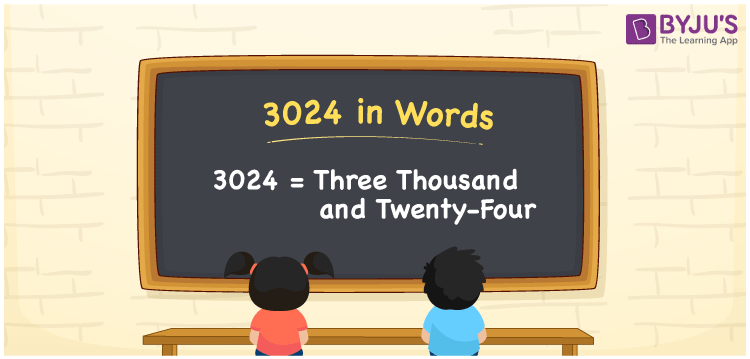# 3024 in Words

3024 in words is Three Thousand and Twenty-Four. For example, if you purchased a water geyser worth Rs. 3024, then you can say, “I purchased a water geyser worth Rupees Three Thousand and Twenty-Four”. 3024 is a cardinal number as it denotes a specific amount. In this article, we will learn how to convert the number 3024 into words with the help of a place value table.

 3024 in Words Three Thousand and Twenty-Four Three Thousand and Twenty-Four in numerical form 3024

## 3024 in English Words

We write the numbers in words with the help of the English alphabet. Hence, we can write the number 3024 in English as Three Thousand and Twenty-Four.## How to Write 3024 in Words?

We can write the number 3024 into words using a place value chart with ease. The place value chart for the number 3024 is given below.

 Thousands Hundreds Tens Ones 3 0 2 4

Hence, we can write the expanded form as:

3 x Thousand + 0 x Hundred + 2 x Ten + 4 x One

= 3 x 1000 + 0 x 100 + 2 x 10 + 4 x 1

= 3000 + 0 + 20 + 4

= 3000 + 20 + 4

= 3024

= Three Thousand and Twenty-Four

Therefore, 3024 in words is written as Three Thousand and Twenty-Four

Interesting way of writing 3024 in words

3 = Three

30 = Thirty

302 = Three Hundred and Two

3024 = Three Thousand and Twenty-Four

Thus, the word form of the number 3024 is Three Thousand and Twenty-Four

3024 is a natural number that precedes 3025 and succeeds 3023

• 3024 in words – Three Thousand and Twenty-Four
• Is 3024 an odd number? – No
• Is 3024 an even number? – Yes
• Is 3024 a perfect square number? – No
• Is 3024 a perfect cube number? – No
• Is 3024 a prime number? – No
• Is 3024 a composite number? – Yes

## Frequently Asked Questions on 3024 in Words

Q1

### How do you write 3024 in words?

3024 in words is written as Three Thousand and Twenty-Four.
Q2

### What is the value 4000 – 976?

4000 – 976 = 3024. The value of 4000 – 976 is 3024 and in words, it is written as Three Thousand and Twenty-Four.
Q3

### Is 3024 a composite number?

Yes, the number 3024 is a composite number.